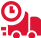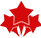OVERALL RATING

Happy Clients

USER REVIEWS

# ASC303 Financial Mathematics Assignment Sample UiTM Malaysia

ASC303 Financial Mathematics is a very important course that all finance students should take. The course covers various financial topics, including the time value of money, annuities, mortgages, and loan repayment. It also teaches students how to use mathematical models to make financial decisions.

This course is essential for understanding the financial concepts used in real-world situations. For example, to understand how a mortgage works, you need to know about compound interest and amortization. And to understand how stocks and bonds are priced, you need to know about the present and future values.

Overall, ASC303 Financial Mathematics is a very informative course that will help finance students better understand the world of finance.

## Get a Solved Assignment sample for ASC303 Financial Mathematics Course

If you are searching for ASC305 Introduction To Actuarial Mathematics Assignment Help services, then your search ends here. Malaysiaassignmenthelp.com is the most trustworthy online academic service provider organization in the industry today. Our tutors hold vast experience and knowledge in this field of study and can guide you with all your university assignments, essays, research papers, case studies, term papers, and dissertations.

In this course, we are discussing some assignment briefs. These are:

### Assignment Brief 1: Describe the concept of the bond as an investment instrument and apply the fundamental concepts in valuing bonds and also in determining the rate of return.

The bond is a debt investment instrument in which a borrower (issuer) promises to make periodic interest payments and to repay the principal at maturity. The bondholder lends money to the issuer in exchange for these periodic payments.

The key concepts in valuing bonds are the present value of future cash flows and the risk premium. The present value of future cash flows is determined by discounting those cash flows back to the present using a rate that reflects the riskiness of the investment. The higher the risk, the higher the required rate of return.

The bond’s rate of return is also affected by its risk premium, which is simply the extra yield that investors demand as compensation for investing in more risky security. This extra yield is called the “default risk premium” and is determined by a number of factors, including the issuer’s creditworthiness.

To calculate the rate of return on a bond, we first need to calculate its yield to maturity. The yield to maturity (YTM) is the rate of return that an investor will earn if he or she holds the bond to maturity and receives all the interest payments and the principal repayment.

To calculate the YTM, we need to discount the bond’s cash flows back to the present using a rate that reflects the bond’s riskiness. The higher the risk, the higher the required rate of return.

The formula for calculating the YTM is as follows:

YTM = [(FV/P)^(1/n)] – 1

where:

FV = face value of the bond

P = price of the bond

n = number of years to maturity

For example, let’s say that you purchase a bond with a face value of \$1,000 for \$950. The bond has a coupon rate of 5% and will mature in 10 years. To calculate the YTM, we would use the following formula:

YTM = [(1,000/950)^(1/10)] – 1 = 0.0526 or 5.26%

The yield to maturity is simply the rate of return that an investor will earn if he or she holds the bond to maturity and receives all the interest payments and the principal repayment.

### Assignment Brief 2: Apply those concepts in calculating present and accumulated values for various streams of cash flows.

When calculating the present value of a series of cash flows, you need to consider two things: the time value of money (the fact that money today is worth more than the same amount tomorrow) and the uncertainty or riskiness of those cash flows.

There are a variety of formulas for calculating present and accumulated values, but all of them take into account these two factors. The most common formula for calculating the present value of a series of cash flows is called the net Present Value (NPV) formula.

The NPV formula takes into account both the time value of money and the uncertainty or riskiness of those cash flows. It calculates how much money you would need to invest today in order to receive all those future cash flows.

The NPV formula is as follows:

NPV = CF1/(1+r)^1 + CF2/(1+r)^2 + … + CFn/(1+r)^n

where:

CF = cash flow

r = discount rate or required rate of return

n = number of periods

For example, let’s say that you are considering investing in a new project. The project will require an initial investment of \$1,000 today and will generate cash flows of \$500 at the end of each of the next five years. The required rate of return on this project is 10%.

To calculate the NPV of this project, we would use the following formula:

NPV = 1,000/(1+0.10)^1 + 500/(1+0.10)^2 + … + 500/(1+0.10)^5

This formula would give us an NPV of \$2,079.72. This means that if you invest \$1,000 today, you would receive cash flows of \$2,079.72 over the next five years.

When considering whether or not to invest in a project, you should always look at the NPV. If the NPV is positive, then you should go ahead with the investment. If the NPV is negative, then you should not go ahead with the investment.

The NPV formula is a powerful tool, but it does have one limitation. It only takes into account the time value of money and does not consider the riskiness of those cash flows.

If you want to take into account the riskiness of those cash flows, you can use the Modified Internal Rate of Return (MIRR) formula.

The MIRR formula is as follows:

MIRR = [(CF1/PVIF(r,n))^(1/n) + (CF2/PVIF(r,n))^(1/n) + … + (CFn/PVIF(r,n))^(1/n)]^(n/(n-1)) – 1

where:

CF = cash flow

PVIF = present value interest factor

r = discount rate or required rate of return

n = number of periods

For example, let’s say that you are considering investing in a new project. The project will require an initial investment of \$1,000 today and will generate cash flows of \$500 at the end of each of the next five years. The required rate of return on this project is 10%.

To calculate the MIRR of this project, we would use the following formula:

MIRR = [(1,000/PVIF(0.10,5))^(1/5) + (500/PVIF(0.10,5))^(1/5) + … + (500/PVIF(0.10,5))^(1/5)]^(5/(5-1)) – 1

This formula would give us a MIRR of 11.11%. This means that if you invest \$1,000 today, you would receive cash flows of \$11.11% over the next five years.

### Assignment Task 3: Describe the fundamental concepts and key terms of financial mathematics.

Financial mathematics is the study of mathematical models and principles to aid in the management, analysis, and interpretation of financial data. It encompasses a wide range of topics from investment analysis and portfolio management to insurance and risk management.

Major concepts in financial mathematics include:

• Interest rate (compound interest, simple interest, annuity)
• Time value of money
• Present value
• Future value
• Net present value
• Internal rate of return
• Price elasticity/demand elasticity(forward price/rate, bond price/yield)

And several key terms are hedging, option pricing models(Black–Scholes), crisis management methods(VaR), financial econometrics(Modelling techniques), and portfolio optimization.

### Assignment Task 4: Apply the key procedure of financial mathematics such as determining equivalent measures of interest, discounting, accumulating, determining yield rates, estimating the rate of return on funds, and amortization.

Financial mathematics is the study of mathematical techniques that are commonly used in financial markets. It is an interdisciplinary field that draws from algebra, probability, and statistics.

Some key procedures in financial mathematics include determining equivalent measures of interest, discounting and accumulating cash flows, estimating yield rates, and constructing hedges. These techniques are important for pricing financial instruments, managing risk, and making investment decisions.

Determining equivalent measures of interest is important for comparing different investment options. Discounting cash flows is a way to account for the time value of money when making investment decisions. Estimating yield rates helps investors compare the potential return on different investments. And constructing hedges can help mitigate risks associated with holding a position in a financial instrument.

## Our professional’s Assignment Experts will fulfill all your assignment writing regarding needs!

If you are searching for an assignment helper Malaysia, you have come to the right place. Malaysia Assignment Help is a professional assignment writing service that can provide you with high-quality and well-written assignments. We have a team of OUM assignment helper who is experts in their respective fields and can provide you with the help you need.

Our thesis writing services offer you the opportunity to buy a custom-written thesis, which will be written by our team of experts. We have a team of professional writers who have extensive experience in writing dissertations and thesis. You can also get civil engineering homework help from our team of experts.

You can also hire professional case study writers from us. We have a team of experienced and qualified writers who can write an effective case study for you. Our writers have extensive experience in writing case studies and can provide you with the help you need. Hurry up and order now!

## Private and Confidential

Yours all information is private and confidential; it is not shared with any other party. So, no one will know that you have taken help for your Academic paper from us.

Online Exam & Assignment Writing Services50000+ Orders Delivered4.9/5
5 Star Rating

Confidential & Secure

Group Assignment Help

Online Exam -Test & Quiz

Cheapest Price Quote

Diploma & Certificate Levels

Semester & FYP Papers

Summative & Individual

GBA & Reflective

Last Minute Assistance

##### CSC799 Usability Engineering UITM Assignment Example Malaysia
Usability engineering is the study of designing interfaces that are easy to use. These methods can be applied at any stage in software development, and they're especially useful when it…
Computer & It
##### FAR110 Financial Accounting 1 Assignment Example UiTM Malaysia
The FAR110 Financial Accounting 1 assignment is a very important and difficult one because it involves learning the basic concepts of financial accounting. In order to be successful, you will…
Accounting

UP TO 15 % DISCOUNT

Instant Paper Writing Services by Native Malaysia Writers

Plagiarism Free Solutions
100% Original Work
24*7 Online Assistance
Native PhD Experts
Hire a Writer Now